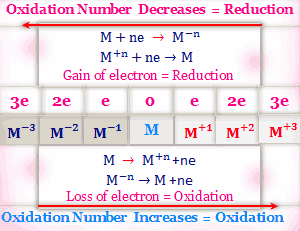# Oxidation reduction reactions

### Oxidation number and concept

The oxidation number of CH4, CH3Cl, CH2Cl2, CHCl3, CCl4 and CO2 are -4, -2, 0, +2, +4 and +4 respectively. In H2, the oxidation number of hydrogen is zero but in H2O it is +1. Similarly, magnesium in the elementary state has a zero oxidation number but in MgCl2, the oxidation number is +2.From the above, we can define Oxidation and Reduction according to the Oxidation number increase or decrees,

#### Oxidation

Oxidation may be defined as a process in which an increase in oxidation number by atoms or ions occurs. A reagent which can increase the oxidation number of an element or ion is called an oxidizing agent.

#### Reduction

The reduction is a process in which a decrease in oxidation number by atoms or ions occurs. A reagent that lowers the oxidation number of an element or ion is called a reducing agent.

#### Representation of oxidation and reduction

Oxidation and reduction are always found to go hand to hand during a redox reaction. Whenever an element or a compound is oxidized, another element or another compound must be simultaneously reduced.An oxidant is reduced and simultaneously the reductant is oxidized.Oxidation number and oxidation-reduction

H2S + Cl2 → 2HCl + S

1. Magnesium metal burns in oxygen to produce magnesium oxide: Before the reaction oxidation number of magnesium and oxygen both zero. But after the reaction, the oxidation number of magnesium and oxygen is +2 and -2 respectively. Thus the oxidation number of magnesium is increases and the oxidation number of oxygen is decreased. So magnesium is oxidized and oxygen is reduced.
Mg + O2 → 2MgO
2. The reaction between Sodium and Chlorine: From the same way, we can explain this reaction. Here oxidation number of Sodium and Chlorine is zero before the reaction and +1 and -1 after the reaction. Thus sodium oxidized and chlorine reduced.
Na + Cl2 → 2NaCl

Problem

Why sulfur dioxide has properties of oxidation and reduction?

• This can be explained by the oxidation state of sulfur. The oxidation numbers of sulfur in the compounds H

2

• S, SO

2

• , and SO

3

are -2, +4 and +6 respectively. Thus the highest oxidation state of sulfur is +6 and the lowest is -2.
• The oxidation state of the free sulfur element is 0. In SO

2

, the oxidation state of sulfur is +4, this oxidation state is the middle of 0 and +6.

Thus, the oxidation state can increases from +4 to +6 and decreases from +4 to 0.

SO₂ + H₂S → 2H₂O + 3S

Previous articleLewis concept of acids and bases
Next articleRutherford model of atom